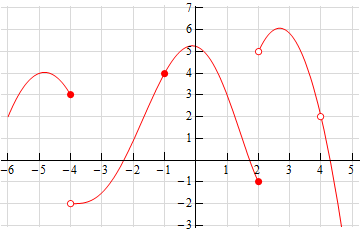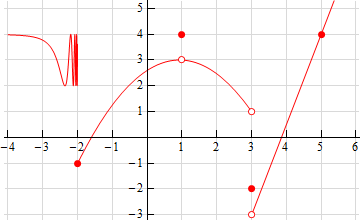Paul's Online Notes
Home / Calculus I / Limits / One-Sided Limits
Show Mobile Notice Show All Notes Hide All Notes
Mobile Notice
You appear to be on a device with a "narrow" screen width (i.e. you are probably on a mobile phone). Due to the nature of the mathematics on this site it is best views in landscape mode. If your device is not in landscape mode many of the equations will run off the side of your device (should be able to scroll to see them) and some of the menu items will be cut off due to the narrow screen width.

### Section 2.3 : One-Sided Limits

1. Below is the graph of $$f\left( x \right)$$. For each of the given points determine the value of $$f\left( a \right)$$, $$\mathop {\lim }\limits_{x \to {a^{\, - }}} f\left( x \right)$$, $$\mathop {\lim }\limits_{x \to {a^{\, + }}} f\left( x \right)$$, and $$\mathop {\lim }\limits_{x \to a} f\left( x \right)$$. If any of the quantities do not exist clearly explain why.
1. $$a = - 4$$
2. $$a = - 1$$
3. $$a = 2$$
4. $$a = 4$$
Solution2. Below is the graph of $$f\left( x \right)$$. For each of the given points determine the value of $$f\left( a \right)$$, $$\mathop {\lim }\limits_{x \to {a^{\, - }}} f\left( x \right)$$, $$\mathop {\lim }\limits_{x \to {a^{\, + }}} f\left( x \right)$$, and $$\mathop {\lim }\limits_{x \to a} f\left( x \right)$$. If any of the quantities do not exist clearly explain why.
1. $$a = - 2$$
2. $$a = 1$$
3. $$a = 3$$
4. $$a = 5$$
Solution3. Sketch a graph of a function that satisfies each of the following conditions. $\mathop {\lim }\limits_{x \to {2^{\, - }}} f\left( x \right) = 1\hspace{0.75in}\mathop {\lim }\limits_{x \to {2^{\, + }}} f\left( x \right) = - 4\hspace{0.75in}f\left( 2 \right) = 1$ Solution
4. Sketch a graph of a function that satisfies each of the following conditions. $\begin{array}{ccl}\mathop {\lim }\limits_{x \to {3^{\, - }}} f\left( x \right) = 0 & \hspace{0.5in}\mathop {\lim }\limits_{x \to {3^{\, + }}} f\left( x \right) = 4 & \hspace{0.5in}f\left( 3 \right){\mbox{ does not exist}}\\ \mathop {\lim }\limits_{x \to - 1} f\left( x \right) = - 3 & \hspace{0.5in} f\left( { - 1} \right) = 2 & \end{array}$ Solution